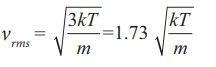Home | | Physics 11th std | Summary - Physics: Kinetic Theory of Gases

# Summary - Physics: Kinetic Theory of Gases

Kinetic theory explains the microscopic origin of macroscopic parameters like temperature, pressure.

SUMMARY

Kinetic theory explains the microscopic origin of macroscopic parameters like temperature, pressure.

The pressure exerted on the walls of gas container is due to the momentum imparted by the gas molecules on the walls.

The pressure. The pressure is directly proportional to the number density, mass of molecule and mean square speed.

The temperature of a gas is a measure of the average translational kinetic energy per molecule of the gas. The average kinetic energy per molecule is directly proportional to absolute temperature of gas and independent of nature of molecules.

The pressure is also equal to 2/3 of internal energy per unit volume.

The rms speed of gas molecules = v  =The average speed of gas moleculesThe most probable speed of gas molecules vmpAmong the speeds vrms is the largest and vmp is the least

vrms > v >vmp

The number of gas molecules in the range of speed v to v+dv is given by Maxwell-Boltzmann distributionThe minimum number of independent coordinates needed to specify the position and configuration of a thermodynamical system in space is called the degrees of freedom of the system. If a sample of gas has N molecules, then the total degrees of freedom f = 3N. If there are q number of constraints then total degrees of freedom f = 3N-q.

For a monoatomic molecule, f = 3

For a diatomic molecule (at normal temperature), f = 5

For a diatomic molecule (at high temperature), f = 7

For a diatomic molecule (at high temperature), f = 7

For a triatomic molecule (linear type), f = 7

For a triatomic molecule (non-linear type), f = 6

The average kinetic energy of sample of gas is equally distributed to all the degrees of freedom. It is called law of equipartition of energy. Each degree of freedom will get 1/2 kT energy.

The ratio of molar specific heat at constant pressure and constant volume of a gasFor

Monoatomic molecule: 1.67

Diatomic molecule (Normal temperature) : 1.40

Diatomic molecule (High temperature): 1.28

Triatomic molecule (Linear type): 1.28.

Triatomic molecule (Non-linear type): 1.33

The mean free path λ = kT / ( √ 2πd2p) . The mean free path is directly proportional to temperature and inversely proportional to size of the molecule and pressure of the molecule

The Brownian motion explained by Albert Einstein is based on kinetic theory. It proves the reality of atoms and molecules.

Study Material, Lecturing Notes, Assignment, Reference, Wiki description explanation, brief detail
11th Physics : UNIT 9 : Kinetic Theory of Gases : Summary - Physics: Kinetic Theory of Gases |